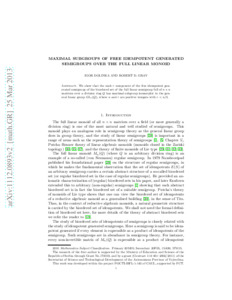# Maximal subgroups of free idempotent generated semigroups over the full linear monoid

Dolinka, Igor and Gray, Robert D. (2014) Maximal subgroups of free idempotent generated semigroups over the full linear monoid. Transactions of the American Mathematical Society, 366. pp. 419-455. ISSN 0002-9947Preview
PDF (GrayTAMSArxivPostPrint) - Submitted Version
We show that the rank $r$ component of the free idempotent generated semigroup of the biordered set of the full linear semigroup full of $n \times n$ matrices over a division ring $Q$ has maximal subgroup isomorphic to the general linear group $GL_r(Q)$, where $n$ and $r$ are positive integers with $r < n/3$.View Item